Anzeige

# Algorithm and flowchart

23. Apr 2016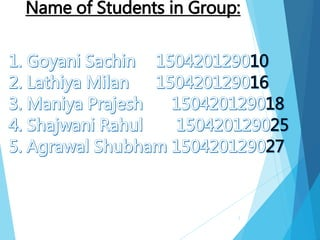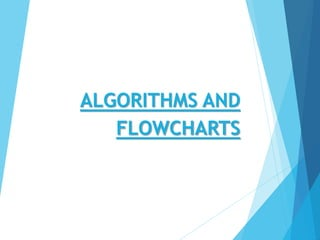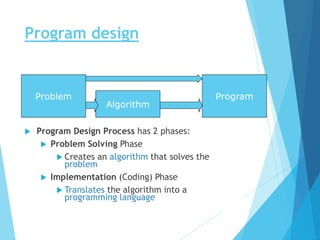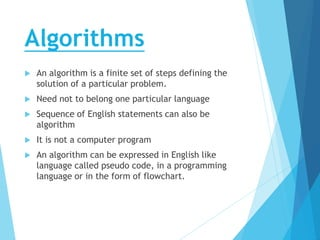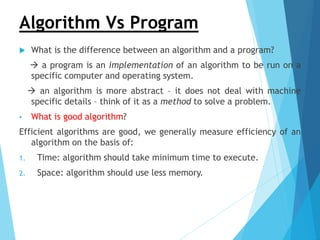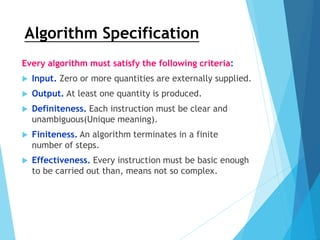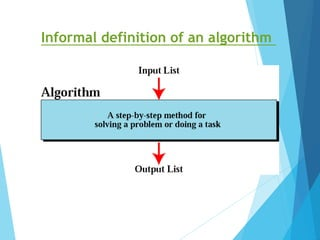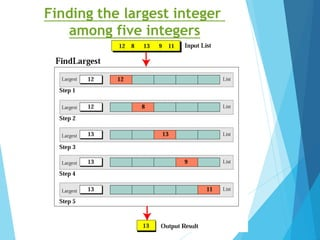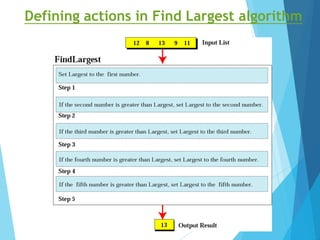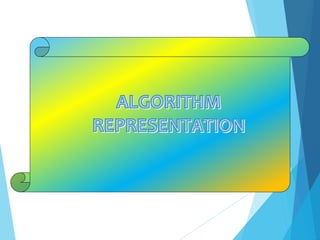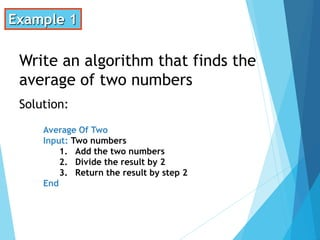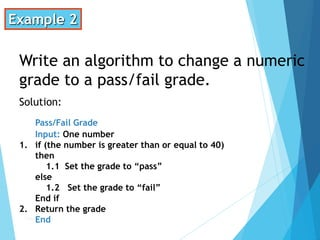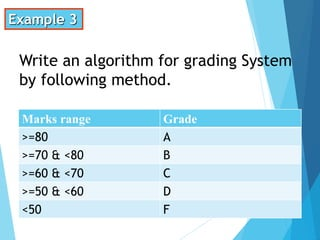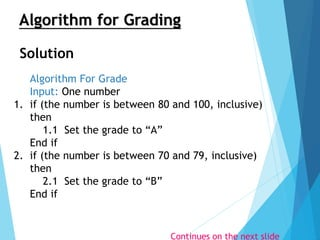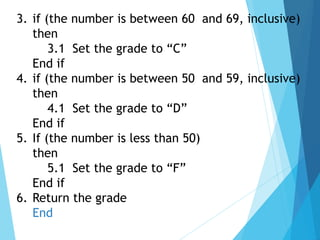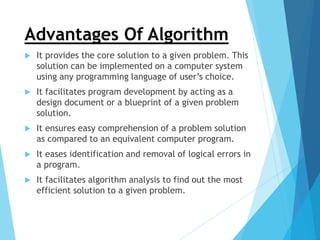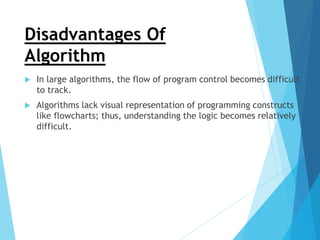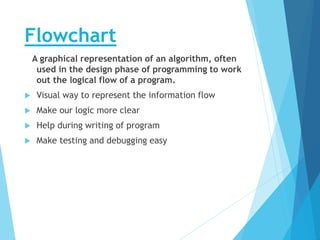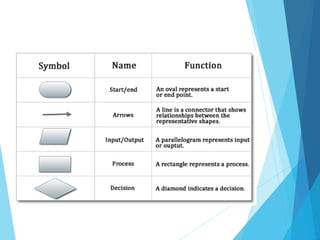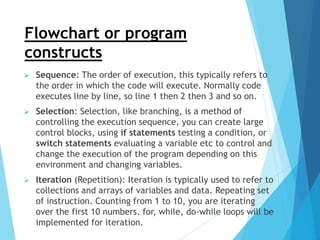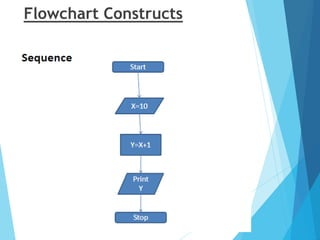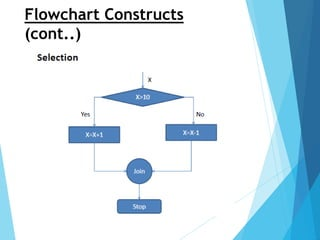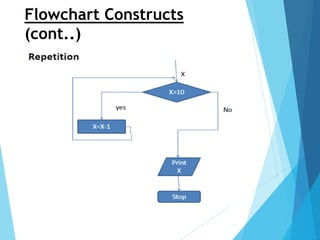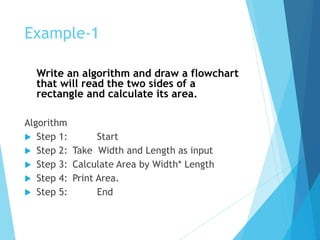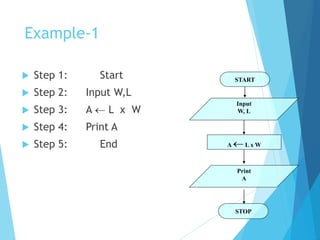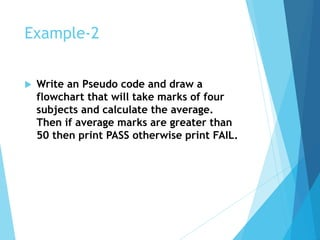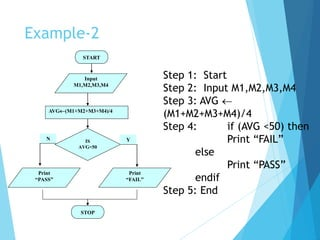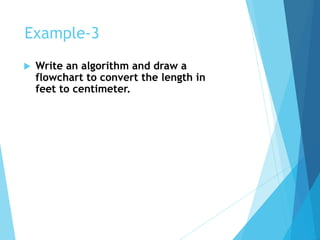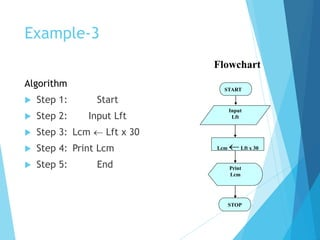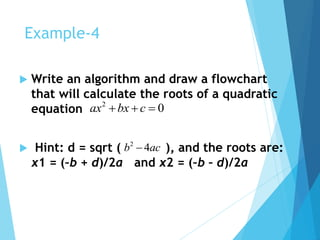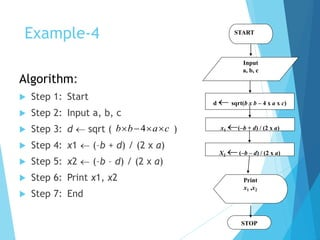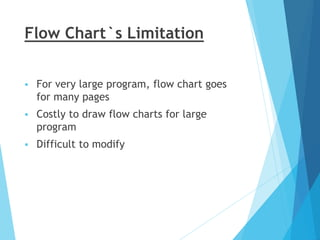1 von 34
Anzeige

### Algorithm and flowchart

1. 1 Subject : C.P.U Topic: Algorithms and Flowchart
2. 2 Name of Students in Group:
3. ALGORITHMS AND FLOWCHARTS
4. Program design  Program Design Process has 2 phases:  Problem Solving Phase  Creates an algorithm that solves the problem  Implementation (Coding) Phase  Translates the algorithm into a programming language 4 Algorithm Problem Program
5. Algorithms  An algorithm is a finite set of steps defining the solution of a particular problem.  Need not to belong one particular language  Sequence of English statements can also be algorithm  It is not a computer program  An algorithm can be expressed in English like language called pseudo code, in a programming language or in the form of flowchart. 5
6. Algorithm Vs Program  What is the difference between an algorithm and a program?  a program is an implementation of an algorithm to be run on a specific computer and operating system.  an algorithm is more abstract – it does not deal with machine specific details – think of it as a method to solve a problem. • What is good algorithm? Efficient algorithms are good, we generally measure efficiency of an algorithm on the basis of: 1. Time: algorithm should take minimum time to execute. 2. Space: algorithm should use less memory. 6
7. Algorithm Specification Every algorithm must satisfy the following criteria:  Input. Zero or more quantities are externally supplied.  Output. At least one quantity is produced.  Definiteness. Each instruction must be clear and unambiguous(Unique meaning).  Finiteness. An algorithm terminates in a finite number of steps.  Effectiveness. Every instruction must be basic enough to be carried out than, means not so complex. 7
8. 8 Informal definition of an algorithm
9. 9 Finding the largest integer among five integers
10. 10 Defining actions in Find Largest algorithm
11. 11
12. 12 Example 1 Write an algorithm that finds the average of two numbers Solution: Average Of Two Input: Two numbers 1. Add the two numbers 2. Divide the result by 2 3. Return the result by step 2 2 End
13. Group 13 Example 2 Write an algorithm to change a numeric grade to a pass/fail grade. Solution: Pass/Fail Grade Input: One number 1. if (the number is greater than or equal to 40) then 1.1 Set the grade to “pass” else 1.2 Set the grade to “fail” End if 2. Return the grade End
14. 14 Example 3 Write an algorithm for grading System by following method. Marks range Grade >=80 A >=70 & <80 B >=60 & <70 C >=50 & <60 D <50 F
15. 15 Algorithm For Grade Input: One number 1. if (the number is between 80 and 100, inclusive) then 1.1 Set the grade to “A” End if 2. if (the number is between 70 and 79, inclusive) then 2.1 Set the grade to “B” End if Algorithm for Grading Continues on the next slide Solution
16. 16 3. if (the number is between 60 and 69, inclusive) then 3.1 Set the grade to “C” End if 4. if (the number is between 50 and 59, inclusive) then 4.1 Set the grade to “D” End if 5. If (the number is less than 50) then 5.1 Set the grade to “F” End if 6. Return the grade End
17. Advantages Of Algorithm  It provides the core solution to a given problem. This solution can be implemented on a computer system using any programming language of user’s choice.  It facilitates program development by acting as a design document or a blueprint of a given problem solution.  It ensures easy comprehension of a problem solution as compared to an equivalent computer program.  It eases identification and removal of logical errors in a program.  It facilitates algorithm analysis to find out the most efficient solution to a given problem. 17
18. Disadvantages Of Algorithm  In large algorithms, the flow of program control becomes difficult to track.  Algorithms lack visual representation of programming constructs like flowcharts; thus, understanding the logic becomes relatively difficult. 18
19. Flowchart A graphical representation of an algorithm, often used in the design phase of programming to work out the logical flow of a program.  Visual way to represent the information flow  Make our logic more clear  Help during writing of program  Make testing and debugging easy 19
20. 20
21. Flowchart or program constructs  Sequence: The order of execution, this typically refers to the order in which the code will execute. Normally code executes line by line, so line 1 then 2 then 3 and so on.  Selection: Selection, like branching, is a method of controlling the execution sequence, you can create large control blocks, using if statements testing a condition, or switch statements evaluating a variable etc to control and change the execution of the program depending on this environment and changing variables.  Iteration (Repetition): Iteration is typically used to refer to collections and arrays of variables and data. Repeating set of instruction. Counting from 1 to 10, you are iterating over the first 10 numbers. for, while, do-while loops will be implemented for iteration. 21
22. Flowchart Constructs 22
23. Flowchart Constructs (cont..) 23
24. 24 Flowchart Constructs (cont..)
25. Example-1 Write an algorithm and draw a flowchart that will read the two sides of a rectangle and calculate its area. Algorithm  Step 1: Start  Step 2: Take Width and Length as input  Step 3: Calculate Area by Width* Length  Step 4: Print Area.  Step 5: End 25
26. Example-1  Step 1: Start  Step 2: Input W,L  Step 3: A  L x W  Step 4: Print A  Step 5: End 26 START Input W, L A L x W STOP Print A
27. Example-2  Write an Pseudo code and draw a flowchart that will take marks of four subjects and calculate the average. Then if average marks are greater than 50 then print PASS otherwise print FAIL. 27
28. Example-2 28 Step 1: Start Step 2: Input M1,M2,M3,M4 Step 3: AVG  (M1+M2+M3+M4)/4 Step 4: if (AVG <50) then Print “FAIL” else Print “PASS” endif Step 5: End START Input M1,M2,M3,M4 AVG(M1+M2+M3+M4)/4 IS AVG<50 STOP YN Print “PASS” Print “FAIL”
29. Example-3  Write an algorithm and draw a flowchart to convert the length in feet to centimeter. 29
30. Example-3 Algorithm  Step 1: Start  Step 2: Input Lft  Step 3: Lcm  Lft x 30  Step 4: Print Lcm  Step 5: End 30 START Input Lft Lcm Lft x 30 Print Lcm STOP Flowchart
31. Example-4  Write an algorithm and draw a flowchart that will calculate the roots of a quadratic equation  Hint: d = sqrt ( ), and the roots are: x1 = (–b + d)/2a and x2 = (–b – d)/2a 2 0ax bx c   2 4b ac 31
32. Example-4 Algorithm:  Step 1: Start  Step 2: Input a, b, c  Step 3: d  sqrt ( )  Step 4: x1  (–b + d) / (2 x a)  Step 5: x2  (–b – d) / (2 x a)  Step 6: Print x1, x2  Step 7: End 4b b a c    32 START Input a, b, c d  sqrt(b x b – 4 x a x c) Print x1 ,x2 STOP x1 (–b + d) / (2 x a) X2 (–b – d) / (2 x a)
33. Flow Chart`s Limitation  For very large program, flow chart goes for many pages  Costly to draw flow charts for large program  Difficult to modify 33

1. 5
Anzeige